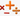## 最小无法表达的正整数

Mon, 27th January 2020Edit on Github加减乘除

# 问题提出

2008年8月mathe提问:

n=1,那么显然只能表示1,所以结果是2.
n=2,由于$1=2-1,2=2\times 1,3=2+1$,4无法表示所以结果是4
n=3,由于$1=\frac{2+1}3,2=3+1-2,3=3\times (2-1),4=3+2-1,5=3\times 2-1,6=3\times 2\times1,7=3\times 2+1,8=(3+1)\times 2,9=3\times (2+1)$,10无法表示,所以结果为10.

# 问题进展

1=(3+2-1)/4
2 = (2-1) + (4-3)
3 = 4 - (1 + 2) / 3
4 = (3 - 1) + (4 - 2)
6 = 1 + 3 + 4 - 2
8 = 2 + 3 + 4 - 1
10 = 1 + 2 + 3 + 4
7 = (1 + 2 + 4) * 1
5 = (2 - 3 + 4) * 1
9 = (2 + 3 + 4) * 1
11 = 2 * 4 + 1 * 3
12 = 2 * 4 + 1 + 3
13 = 3 * 4 + 2 - 1
15 = 3 * 4 + 2 + 1
14 = 1 * 3 * 4 + 2
16 = (1 + 3) * 4 + 2
17 = (1 + 4) * 3 + 2
18 = (4 - 1) * 3 * 2
19 = (4 + 2) * 3 + 1
20 = (3 * 2 - 1) * 4
21 = ( 4 + 2 + 1) * 3
22 = (4 * 3 - 1) * 2
23 = 4 * 3 * 2 - 1
24 = (4 + 2) * (1 + 3)
25 = (4 + 1) * (2 + 3)
26 = (4 * 3 + 1) * 2
27 = (4 * 2 + 1) * 3
28 = (2 * 3 + 1)  * 4

kenmark认为应该$a[n]\gt \frac{n(n+1)}2$.

mathe提议应该使用加减乘除产生的表达式计数中的方法，可将穷举数目降低到$2,894,532,443,154$

mathe发现A060315已经计算到n=10了。 mathe也提供了他的可以计算到n=10的代码
mathe介绍他的实现主要是要避免产生等价的表达式，但是每个表达式都要从头开始计算。当然应该还可以做一些优化，但是从复杂度看，不会有本质的改善。而如果将宏CACHELIMIT定义成非零的值。那么那些操作数数目不超过CACHELIMIT的子表达式的结果会被缓存起来（结果重复的会被合并），从而表达式其他部分发生更新时这个部分不需要重复计算。这个方法可以用来提高一些计算速度。但是CACHE_LIMIT不能太大，不然内存消耗受不了。当然程序采用分数运算也降低了速度，但是保证了正确性.

r=10
r=29
r=76
r=284
r=1413
r=7187
r=38103
r=231051
r=1765186
r=10539427

Github